You are here: Home > Products > Network Monitor (Pro) > User Manual > Network Monitoring Checks > ARP Monitoring, MAC address monitoring

# Expression Check (Counting Formulas)

With this check, you can calculate values counted with formulas using other checks' values. For example, some SNMP performance parameters can be counted using two or more SNMP variables. The free disk space is a parameter of this type. The program can receive this value in some "units" instead of bytes from a network device. You need to get size of these units in another check because one monitoring check can only get and monitor one variable. The expression check can help in this case and count the free disk space in regular bytes.

You can also calculate performance counters in per cent using the maximum possible and current values with this check.

One of possible appliances is the current network bandwidth monitoring. You can get the previous traffic count, the current count, and time between these counts using special substitution keys. The simple formula "(Current-Previous)/Time" allows you to count the current bandwidth in bytes per second.

The expression check supports a comprehensive set of operators, keys, and functions for creating any type of formula.

Substitution keys:

CHECK1, CHECK2... - current monitored values for checks in the list

PREV1, PREV2... - previous values for checks in the list (values received during the previous check polling).

TIME1, TIME2... - time between the current and previous monitoring checks (1, 2... - the check numbers in the list)

Possible operators:

• - subtraction
• * multiplication
• / division
• ^ exponentiation (example: 2^8)
• MOD - remainder (example: 10MOD3, result = 1)
• DIV - integer division (example: 10DIV3, result = 3)

Possible functions:

• Trigonometric functions: COS (cosine), SIN (sinus), SINH (hyperbolic sinus), COSH (hyperbolic cosine), TAN (tangent), COTAN (cotangent), ARCTAN (arctangent), ARG (). Example: COS(CHECK1))
• Logarithmic functions: EXP, LN, LOG10, LOG2, LOGN
• SQRT (square root), SQR (square), POWER (involution, example POWER(2,3)), INTPOWER (involution X to integer power of Y)
• MIN (minimum), MAX (maximum), ABS (magnitude), TRUNC (integer part), INT (the same as TRUNC), CEIL, FLOOR
• HEAV (heav(x)=1 for x>0, and =0 for x<=0)
• SIGN (sign(x)=1 for x>1, and=0 for x=0, =-1 for x<0)
• ZERO (zero(x)=0 for x=0, and =1 for x<>0)
• PH (ph(x) = x - 2*pi*round(x/2/pi))
• RND (rnd(x) = int(x) * Random)
• RANDOM (random(X) = Random; X is not used)

Brackets are allowed (maximum nesting is 20).

No spaces are allowed.

Specify an expression using operators, keys, and functions in the Formula string and click the Get button for testing.

Requirements: Windows XP/Vista/7/8.1/10/11, Server 2003/2008/2012/2016/2019/2022 supported.Network Inventory Explorer
Inventory Hardware and Software on Network Computers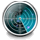Network Monitor
Monitor Network Servers, Services, Managed Switches, etc.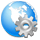LANState
Monitor and Manage Network Hosts on a Visual Map, Create Network Maps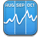Bandwidth Monitor
Monitor Network Bandwidth and the Internet Traffic UsageNetwork Diagram
Create Network Diagrams, Discover Network Topology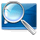Network File Search
Search Files on Network Shares and FTP Servers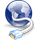Connection Monitor